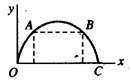Projection Under uniform Acceleration
Question

# There are two values of time for which a projectile is at the same height. The sum of these two times is equal to (T = time of flight of the projectile)

Easy
Solution

##${\mathrm{t}}_{\mathrm{OA}}+{\mathrm{t}}_{\mathrm{OB}}={t}_{BC}+{t}_{OB}=\mathrm{T}$

Get Instant Solutions